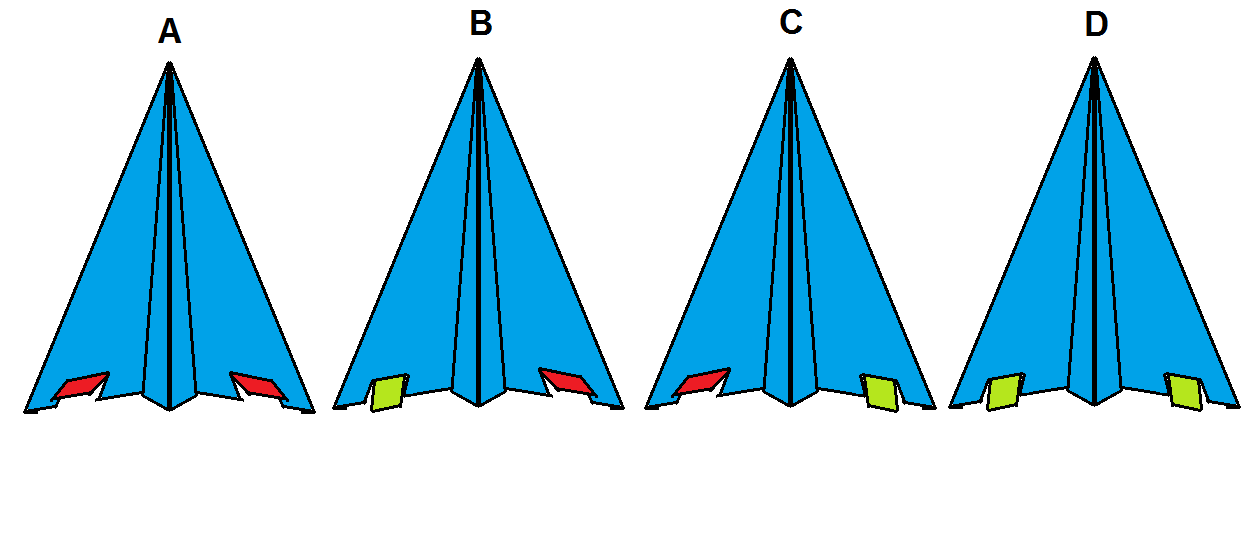# Paper plane dynamicsRakesh has made a generic paper airplane which when launched perfectly, flies straight and lands smoothly on the ground after a while. He gets bored with it and decides to experiment. He makes one cut each on the bottom of each wing and bends the cut piece up or down. If the piece is bent up, it is shown in red, if it is bent down, it is shown in green. Rakesh tests the nature of the flight trajectory of the plane for different configurations of the wings. He makes perfect launches every time. Each configuration is denoted in the diagram.

Finally, he compares the trajectories of each of the scenarios $A,B,C,D$ with the generic unmodified paper plane's trajectory. He marks under each letter, the most suitable number according to the following rules:

1. If the plane deviates left from normal trajectory, mark $1$.

2. If the plane deviates right from normal trajectory, mark $2$.

3. If the plane flies significantly longer than normal, mark $3$.

4. If the plane makes a nose dive, mark $4$.

5. Otherwise, mark $0$.

Rakesh now has a four digit number underneath the letters. What is this number?

×